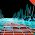## Monday, March 21, 2011

### ARIMA, Forecasting and Python

I ported the R code found on Rob Hyndman's blog into Python + rpy2. The latter package allows calling of R code from Python which we used here to utilize the forecast package. Test R and Python codes can also be found in the zip file below.
```import rpy2.robjects as R
import rpy2.rinterface as rinterface
from rpy2.robjects.packages import importr
import rpy2.robjects.numpy2ri
from rpy2.robjects.vectors import FloatVector, StrVector
import datetime
import numpy as np
forecast = importr("forecast")
R.r.source("arima-fn.R")

x = np.loadtxt("garan.dat",  skiprows=1)
n = len(x)
m = 10
terms = 4

x = np.insert(x, 10, np.nan) # put in a NaN to see what happens
n += 1

print x

R.r.assign('x',x)
R.r.assign('n',n)
R.r.assign('m',m)
R.r.assign('terms',terms)

R.r('fit = Arima(x=x, order=c(2,0,1), xreg=fourier(1:n,terms,m))')
R.r('f = forecast(fit, h=2*m, xreg=fourier(n+1:(2*m),terms,m))')
res = R.r('forecast_results(f)')
for x in res:
print x```

All Code

arima-fourier2.R

forecast_results <- function(res)
{
return (res\$mean)
}

fourier <- function(t,terms,period)
{
print (terms)
n <- length(t)
X <- matrix(,nrow=n,ncol=2*terms)
for(i in 1:terms)
{
X[,2*i-1] <- sin(2*pi*i*t/period)
X[,2*i] <- cos(2*pi*i*t/period)
}
colnames(X) <- paste(c("S","C"),rep(1:terms,rep(2,terms)),sep="")
return(X)
}

library(forecast)

y <- yy[,'price']

n <- length(y)
print (n)
m <- 10 # how many points in the future
terms = 4

fit <- Arima(y, order=c(2,0,1), xreg=fourier(1:n,terms,m))

f = forecast(fit, h=2*m, xreg=fourier(n+1:(2*m),terms,m))

print (forecast_results(f))

plot(f)

arima-fouerier.R

n <- 2000
m <- 200
y <- ts(rnorm(n) + (1:n)%%100/30, f=m)
print (y)
quit()
fourier <- function(t,terms,period)
{
n <- length(t)
X <- matrix(,nrow=n,ncol=2*terms)
for(i in 1:terms)
{
X[,2*i-1] <- sin(2*pi*i*t/period)
X[,2*i] <- cos(2*pi*i*t/period)
}
colnames(X) <- paste(c("S","C"),rep(1:terms,rep(2,terms)),sep="")
return(X)
}

library(forecast)
fit <- Arima(y, order=c(2,0,1), xreg=fourier(1:n,4,m))
f = forecast(fit, h=2*m, xreg=fourier(n+1:(2*m),4,m))
plot(f)

print (y)
print (f)

arima.py

import rpy2.robjects as R
import rpy2.rinterface as rinterface
from rpy2.robjects.packages import importr
import rpy2.robjects.numpy2ri
from rpy2.robjects.vectors import FloatVector, StrVector
import datetime
import numpy as np
forecast = importr("forecast")
R.r.source("arima-fn.R")

x = np.loadtxt("garan.dat",  skiprows=1)
n = len(x)
m = 10
terms = 4

x = np.insert(x, 10, np.nan) # put in a NaN to see what happens
n += 1

print x

R.r.assign('x',x)
R.r.assign('n',n)
R.r.assign('m',m)
R.r.assign('terms',terms)

R.r('fit = Arima(x=x, order=c(2,0,1), xreg=fourier(1:n,terms,m))')
R.r('f = forecast(fit, h=2*m, xreg=fourier(n+1:(2*m),terms,m))')
res = R.r('forecast_results(f)')
for x in res:
print x

garan.dat

price
6.40000
6.33000
6.43000
6.53000
6.48000
6.33000
6.28000
6.33000
6.33000
6.18000
6.28000
6.28000
6.43000
6.48000
6.23000
6.38000
6.33000
6.23000
6.45000
6.28000
6.38000
6.43000
6.45000
6.23000
6.04000
5.84000
5.94000
5.84000
5.95000
5.89000
5.94000
6.00000
6.35000
6.13000
6.38000
6.23000
6.04000
5.79000
5.49000
5.70000

1.Thanks for this! I've had rpy2 installed for a while, but haven't had a chance to learn much about its use yet. Examples like this help a ton.

I had two questions from running your arima.py file; it gave me two errors:

One was that R couldn't find a function forecast_results() in the forecast package? Switching it to summary(f) gave me some neat output and an idea of what was going on, though. Looking in the paper that describes the forecast package (http://www.jstatsoft.org/v27/i03/paper), I couldn't find anything that looked like forecast_results -- was there supposed to be something else there, or am I doing something wrong?

The second error was that I had to add rpy2.robjects.numpy2ri.activate() for rpy2 to know how to convert the numpy array that x was defined as into a suitable R object during the R.r.assign('x', x) line. From what I googled, it sounded like this was sort of recently changed, although I don't know that I was able to follow all the details of the discussion.

2.You installed the forecast package on R right?